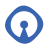#Open Source Glasgow

### Site Tools

events:getting_started_with_godot_in_3d

# Getting Started with Godot in 3D

### Notes for everyone

• Create 3D spatial node, called World
• Add child node, create KinematicBody, called Player
• Add child node to player, type Collision Shape, type capsule
• Make vertical. Transform x by 90 degrees.
• Click capsule shape dropdown, Set radius to 0.75, height to 1.25.
• Add child node to head, type camera, move it to around 0.9 on the Y axis
• Add a child to the player node, add meshinstance with shapre capsule
• Size the capsule to the collision mesh - click on capusulemesh then 0.75 radius, height 1.25 and transform rotate 90 on x
• Add new child now to World, MeshInstance, CubeMesh
• drag below player, then scale to a floor size (20×20 or whatever)
• Click, Mesh, create Trimesh static body
• Save Player as Scene
• Enter Player scene
• edit input map (Project → Project Settings → Input Map)
• add move_forward, move_backward, move_left, move_right and jump
• assign keys (WASD, space)
• Right Click player, attach script, default, template empty
```extends KinematicBody

export var speed = 10
export var acceleration = 5
export var gravity = 0.98
export var jump_power = 30

var velocity = Vector3()

func _physics_process(delta):

var direction = Vector3()
if Input.is_action_pressed("move_forward"):
elif Input.is_action_pressed("move_backward"):

if Input.is_action_pressed("move_left"):
elif Input.is_action_pressed("move_right"):

direction = direction.normalized()
velocity = velocity.linear_interpolate(direction * speed, acceleration * delta)

velocity = move_and_slide(velocity)```
• Test the above code out, now lets add some camera/head controls
```extends KinematicBody

export var speed = 10
export var acceleration = 5
export var gravity = 0.98
export var jump_power = 30
export var mouse_sensitivity = 0.3

var velocity = Vector3()
var camera_x_rotation = 0

func _input(event):
if event is InputEventMouseMotion:
var x_delta = event.relative.y * mouse_sensitivity
if camera_x_rotation + x_delta > -90 and camera_x_rotation + x_delta < 90:
camera_x_rotation += x_delta

func _physics_process(delta):

var direction = Vector3()
if Input.is_action_pressed("move_forward"):
elif Input.is_action_pressed("move_backward"):

if Input.is_action_pressed("move_left"):
elif Input.is_action_pressed("move_right"):

direction = direction.normalized()
velocity = velocity.linear_interpolate(direction * speed, acceleration * delta)

velocity = move_and_slide(velocity)```
`velocity.y += gravity`
```if Input.is_action_just_pressed("jump"):
velocity.y += jump_power```
• Define the floor to fix jumping
```	if Input.is_action_just_pressed("jump") and is_on_floor():
velocity.y += jump_power

velocity = move_and_slide(velocity, Vector3.UP)```
• Lock the mouse to the window
```func _ready():
Input.set_mouse_mode(Input.MOUSE_MODE_CAPTURED)

func _process(delta):
if Input.is_action_just_pressed("ui_cancel"):
Input.set_mouse_mode(Input.MOUSE_MODE_VISIBLE)```
• I think that's as far as we'll get in the first session, I'll make a start on part two though.

### Notes for me

• Axis, blue is Z (left to right generally), green is Y (up and down), X is red, back and forward (depth)
• Explain viewports:
• move mode:
• middle mouse - change position
• shift middle, move around in 3d space
• right mouse - change angle
• rotate mode:
• middle rotate around object
• mouse wheel zoom
• F to focus on object, O to focus on origin
• right mouse, WASD
• Ctrl + Left click for inline help